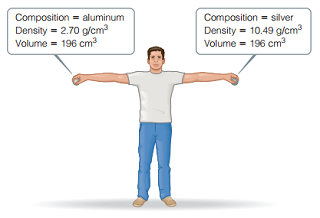# Problem: You may want to reference (Pages 15 - 16) Section 1.4 while completing this problem.Consider the two spheres shown here, one made of silver and the other of aluminum.The force of gravity acting on an object is F = mg, where m is the mass of an object and g is the acceleration of gravity (9.8 m/s2). How much work do you do on the aluminum sphere if you raise it from the floor to a height of 2.1 m?

###### FREE Expert Solution

Calculate the mass of each sphere:

80% (2 ratings)###### Problem Details

You may want to reference (Pages 15 - 16) Section 1.4 while completing this problem.

Consider the two spheres shown here, one made of silver and the other of aluminum.The force of gravity acting on an object is F = mg, where m is the mass of an object and g is the acceleration of gravity (9.8 m/s2). How much work do you do on the aluminum sphere if you raise it from the floor to a height of 2.1 m?

Frequently Asked Questions

What scientific concept do you need to know in order to solve this problem?

Our tutors have indicated that to solve this problem you will need to apply the Internal Energy concept. You can view video lessons to learn Internal Energy. Or if you need more Internal Energy practice, you can also practice Internal Energy practice problems.